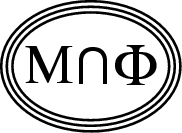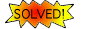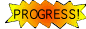Back to
OP page

Combined
List

List by
Contributors

# Exponents and Dimensions

### BREAKTHROUGH RESULTS ON THESE QUESTIONS HAVE BEEN OBTAINED BY Gregory F. Lawler, Oded Schramm, Wendelin Werner, and Stanislav Smirnov (see below)

One does not need to look far in order to find exact predictions, which appear to be correct but whose derivation is beyond the rigorous methods. Following are some examples, involving easily described processes - all in two dimensions -

True or false:
1. The dimension of the self-avoiding walk is dSAW = 4/3
[as implied by   ]   (?)

2. The dimension of the frontier of Brownian motion is also 4/3
[a conjecture of B. Mandelbrot]   (?)TRUE -- proven by Gregory F. Lawler, Oded Schramm, and Wendelin Werner

3. The probability that L simple walks (starting at fixed points) have non-intersecting trajectories decays with time t as const./tz(L), with the exponents
z(L) = (4L2-1)/24        (?)TRUE -- proven in the above work; see also related articles

4. The dimension of the boundaries of the connected clusters of critical percolation models is dHull=7/4  (), coinciding with the dimensions of polymers at the "theta-point", but the dimension of the clusters' external (exposed) perimeter is (again) 4/3   (?)
(Ref.  and references therein.)Proven for site perc. on the triangular lattice by Stanislav Smirnov and Wendelin Werner
Similar exact claims exist for the dimensions of fractal sets, and exponents, associated with the large-scale excitations in many models -- at their critical points. These include: percolation, Ising and Potts spin systems, quantum spin chains, and tilings.

Dimensions and exponents are linked (a point stressed by Mandelbrot, and utilized in ref. ). The relation is easy to understand on a non-rigorous level, though upon closer inspection one may find surprizes, e.g., in the scaling limit the upper-box dimension of the critical percolation clusters does not equal their Hausdorff dimension .

Methods yielding exact predictions include: "Coulomb gas" representation, conformal field theory and "quantum gravity". The subjects are discussed in the excellent compilation . Typically, the analysis incorportes some (rather clever) assumptions about the structure of the scaling limit. An important challenge is to develop the mathematical tools which would permit to derive such information from within the model.

Recent works tying the exact with the rigorous include Ref. , where rigorous relations are established between percolation path-crossing exponents and "Coulomb-gas" exponents, and Ref. , which concerns the non-intersection exponents of Brownian motion.

References:
1. B. Nienhuis. Phys. Rev. lett. 49, 1063 (1982).

2. B. Duplantier: "Random walks and quantum gravity in two dimensions", Phys. Rev. Lett. 81, 5489 (1998) (and earlier work with K.-H. Kwon).

3. H. Saleur and B. Duplantier. Phys. Rev. Lett. 58, 2325 (1987).

4. M. Aizenman, B. Duplantier and A. Aharony: "Path crossing exponents and the external perimeter in 2D percolation", Phys. Rev. Lett. 83, 1359 (1999).

5. M. Aizenman and A. Burchard. "Holder Regularity and Dimension Bounds for Random Curves", Duke Math. Journ. 99, 419 (1999).

6. E. Brezin, J. Zinn-Justin (editors), Fields, Strings and Critical Phenomena (Les Houches 1988), (North Holland 1990) (and references therein).

7. G.F. Lawler and W. Werner. "Intersection exponents for planar Brownian motion", 1998 preprint.